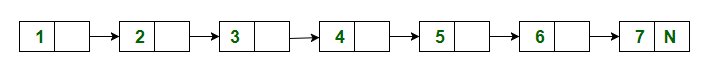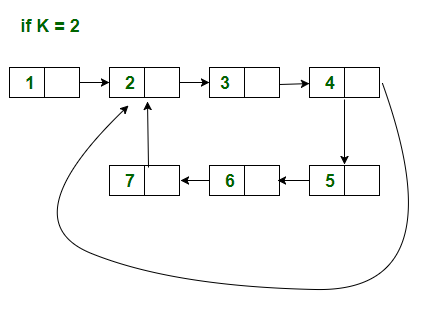# Make a loop at k-th position in a linked list

Given a linked list and a position k. Make a loop at k-th position

Examples:

```Input : Given linked list```

## Recommended: Please try your approach on {IDE} first, before moving on to the solution.

Algorithm
1) Traverse the first linked list till k-th point
2) Make backup of the k-th node
4) Attach the last node with k-th node

## C++

 `// CPP program for making loop at k-th index ` `// of given linked list ` `#include ` `using` `namespace` `std; ` ` `  `/* Link list node */` `struct` `Node { ` `    ``int` `data; ` `    ``struct` `Node* next; ` `}; ` ` `  `/* Function to make loop at k-th elements of ` `linked list */` `void` `makeloop(``struct` `Node** head_ref, ``int` `k) ` `{ ` `    ``// traverse the linked list until loop ` `    ``// point not found ` `    ``struct` `Node* temp = *head_ref; ` `    ``int` `count = 1; ` `    ``while` `(count < k) { ` `        ``temp = temp->next; ` `        ``count++; ` `    ``} ` ` `  `    ``// backup the joint point  ` `    ``struct` `Node* joint_point = temp;  ` ` `  `    ``// traverse remaining nodes ` `    ``while` `(temp->next != NULL)  ` `        ``temp = temp->next; ` ` `  `    ``// joint the last node to k-th element ` `    ``temp->next = joint_point; ` `} ` ` `  `/* Function to push a node */` `void` `push(``struct` `Node** head_ref, ``int` `new_data) ` `{ ` `    ``struct` `Node* new_node = ` `        ``(``struct` `Node*)``malloc``(``sizeof``(``struct` `Node)); ` `    ``new_node->data = new_data; ` `    ``new_node->next = (*head_ref); ` `    ``(*head_ref) = new_node; ` `} ` ` `  `/* Function to print linked list */` `void` `printList(``struct` `Node* head, ``int` `total_nodes) ` `{ ` `    ``struct` `Node* curr = head; ` `    ``int` `count = 0; ` `    ``while` `(count < total_nodes) { ` `        ``count++; ` `        ``cout << curr->data << ``" "``; ` `        ``curr = curr->next; ` `    ``} ` `} ` ` `  `int` `countNodes(Node *ptr) ` `{ ` `    ``int` `count = 0; ` `    ``while` `(ptr != NULL) ` `    ``{ ` `        ``ptr = ptr->next; ` `        ``count++; ` `    ``} ` `    ``return` `count; ` `} ` ` `  `/* Driver program to test above function*/` `int` `main() ` `{ ` `    ``// Create a linked list 1->2->3->4->5->6->7 ` `    ``struct` `Node* head = NULL; ` `    ``push(&head, 7); ` `    ``push(&head, 6); ` `    ``push(&head, 5); ` `    ``push(&head, 4); ` `    ``push(&head, 3); ` `    ``push(&head, 2); ` `    ``push(&head, 1); ` ` `  `    ``// k should be less than the ` `    ``// numbers of nodes ` `    ``int` `k = 4; ` `    ``int` `total_nodes = countNodes(head); ` ` `  `    ``cout << ``"\nGiven list\n"``; ` `    ``printList(head, total_nodes); ` ` `  `    ``makeloop(&head, k); ` ` `  `    ``cout << ``"\nModified list\n"``; ` `    ``printList(head, total_nodes); ` `    ``return` `0; ` `} `

## Java

 `// Java program for making loop at k-th index  ` `// of given linked list  ` `class` `GFG ` `{ ` `     `  `// Link list node / ` `static` `class` `Node ` `{  ` `    ``int` `data;  ` `    ``Node next;  ` `}  ` ` `  `// Function to make loop at k-th elements of  ` `//linked list / ` `static` `Node makeloop( Node head_ref, ``int` `k)  ` `{  ` `    ``// traverse the linked list until loop  ` `    ``// point not found  ` `    ``Node temp = head_ref;  ` `    ``int` `count = ``1``;  ` `    ``while` `(count < k) ` `    ``{  ` `        ``temp = temp.next;  ` `        ``count++;  ` `    ``}  ` ` `  `    ``// backup the joint point  ` `    ``Node joint_point = temp;  ` ` `  `    ``// traverse remaining nodes  ` `    ``while` `(temp.next != ``null``)  ` `        ``temp = temp.next;  ` ` `  `    ``// joint the last node to k-th element  ` `    ``temp.next = joint_point;  ` `    ``return` `head_ref; ` `}  ` ` `  `// Function to push a node / ` `static` `Node push( Node head_ref, ``int` `new_data)  ` `{  ` `    ``Node new_node = ``new` `Node();  ` `    ``new_node.data = new_data;  ` `    ``new_node.next = (head_ref);  ` `    ``(head_ref) = new_node;  ` `    ``return` `head_ref; ` `}  ` ` `  `// Function to print linked list / ` `static` `void` `printList( Node head, ``int` `total_nodes)  ` `{  ` `    ``Node curr = head;  ` `    ``int` `count = ``0``;  ` `    ``while` `(count < total_nodes) ` `    ``{  ` `        ``count++;  ` `        ``System.out.print(curr.data + ``" "``);  ` `        ``curr = curr.next;  ` `    ``}  ` `}  ` ` `  `static` `int` `countNodes(Node ptr)  ` `{  ` `    ``int` `count = ``0``;  ` `    ``while` `(ptr != ``null``)  ` `    ``{  ` `        ``ptr = ptr.next;  ` `        ``count++;  ` `    ``}  ` `    ``return` `count;  ` `}  ` ` `  `// Driver code ` `public` `static` `void` `main(String args[]) ` `{  ` `    ``// Create a linked list 1.2.3.4.5.6.7  ` `    ``Node head = ``null``;  ` `    ``head = push(head, ``7``);  ` `    ``head = push(head, ``6``);  ` `    ``head = push(head, ``5``);  ` `    ``head = push(head, ``4``);  ` `    ``head = push(head, ``3``);  ` `    ``head = push(head, ``2``);  ` `    ``head = push(head, ``1``);  ` ` `  `    ``// k should be less than the  ` `    ``// numbers of nodes  ` `    ``int` `k = ``4``;  ` `    ``int` `total_nodes = countNodes(head);  ` ` `  `    ``System.out.print(``"\nGiven list\n"``);  ` `    ``printList(head, total_nodes);  ` ` `  `    ``head = makeloop(head, k);  ` ` `  `    ``System.out.print( ``"\nModified list\n"``);  ` `    ``printList(head, total_nodes);  ` `} ` `}  ` ` `  `// This code is contributed by Arnab Kundu `

## Python3

 `# Python3 program for making loop at k-th index ` `# of given linked list ` `import` `math ` ` `  `# Link list node  ` `class` `Node:  ` `    ``def` `__init__(``self``, data):  ` `        ``self``.data ``=` `data  ` `        ``self``.``next` `=` `None` ` `  `# Function to make loop at k-th elements of ` `#linked list  ` `def` `makeloop(head_ref, k): ` `     `  `    ``# traverse the linked list until loop ` `    ``# point not found ` `    ``temp ``=` `head_ref ` `    ``count ``=` `1` `    ``while` `(count < k): ` `        ``temp ``=` `temp.``next` `        ``count ``=` `count ``+` `1` ` `  `    ``# backup the joint point  ` `    ``joint_point ``=` `temp  ` ` `  `    ``# traverse remaining nodes ` `    ``while` `(temp.``next` `!``=` `None``):  ` `        ``temp ``=` `temp.``next` ` `  `    ``# joint the last node to k-th element ` `    ``temp.``next` `=` `joint_point ` `    ``return` `head_ref ` ` `  `# Function to push a node  ` `def` `push(head_ref, new_data): ` `    ``new_node ``=` `Node(new_data) ` `    ``new_node.data ``=` `new_data ` `    ``new_node.``next` `=` `head_ref ` `    ``head_ref ``=` `new_node ` `    ``return` `head_ref ` ` `  `# Function to print linked list  ` `def` `printList( head,total_nodes): ` `    ``curr ``=` `head ` `    ``count ``=` `0` `    ``while` `(count < total_nodes): ` `        ``count ``=` `count ``+` `1` `        ``print``(curr.data, end ``=` `" "``) ` `        ``curr ``=` `curr.``next` `     `  `def` `countNodes(ptr): ` `    ``count ``=` `0` `    ``while` `(ptr !``=` `None``): ` `        ``ptr ``=` `ptr.``next` `        ``count ``=` `count ``+` `1` `     `  `    ``return` `count ` ` `  `# Driver Code ` `if` `__name__``=``=``'__main__'``: ` `     `  `    ``# Create a linked list 1.2.3.4.5.6.7 ` `    ``head ``=` `None` `    ``head ``=` `push(head, ``7``) ` `    ``head ``=` `push(head, ``6``) ` `    ``head ``=` `push(head, ``5``) ` `    ``head ``=` `push(head, ``4``) ` `    ``head ``=` `push(head, ``3``) ` `    ``head ``=` `push(head, ``2``) ` `    ``head ``=` `push(head, ``1``) ` ` `  `    ``# k should be less than the ` `    ``# numbers of nodes ` `    ``k ``=` `4` `    ``total_nodes ``=` `countNodes(head) ` ` `  `    ``print``(``"Given list"``) ` `    ``printList(head, total_nodes) ` ` `  `    ``makeloop(head, k) ` ` `  `    ``print``(``"\nModified list"``) ` `    ``printList(head, total_nodes) ` `     `  `# This code is contributed by Srathore `

## C#

 `// C# program for making loop at k-th index  ` `// of given linked list  ` `using` `System; ` ` `  `class` `GFG ` `{ ` `     `  `// Link list node / ` `public` `class` `Node ` `{  ` `    ``public` `int` `data;  ` `    ``public` `Node next;  ` `}  ` ` `  `// Function to make loop at k-th elements of  ` `//linked list / ` `static` `Node makeloop( Node head_ref, ``int` `k)  ` `{  ` `    ``// traverse the linked list until loop  ` `    ``// point not found  ` `    ``Node temp = head_ref;  ` `    ``int` `count = 1;  ` `    ``while` `(count < k) ` `    ``{  ` `        ``temp = temp.next;  ` `        ``count++;  ` `    ``}  ` ` `  `    ``// backup the joint point  ` `    ``Node joint_point = temp;  ` ` `  `    ``// traverse remaining nodes  ` `    ``while` `(temp.next != ``null``)  ` `        ``temp = temp.next;  ` ` `  `    ``// joint the last node to k-th element  ` `    ``temp.next = joint_point;  ` `    ``return` `head_ref; ` `}  ` ` `  `// Function to push a node / ` `static` `Node push( Node head_ref, ``int` `new_data)  ` `{  ` `    ``Node new_node = ``new` `Node();  ` `    ``new_node.data = new_data;  ` `    ``new_node.next = (head_ref);  ` `    ``(head_ref) = new_node;  ` `    ``return` `head_ref; ` `}  ` ` `  `// Function to print linked list / ` `static` `void` `printList( Node head, ``int` `total_nodes)  ` `{  ` `    ``Node curr = head;  ` `    ``int` `count = 0;  ` `    ``while` `(count < total_nodes) ` `    ``{  ` `        ``count++;  ` `        ``Console.Write(curr.data + ``" "``);  ` `        ``curr = curr.next;  ` `    ``}  ` `}  ` ` `  `static` `int` `countNodes(Node ptr)  ` `{  ` `    ``int` `count = 0;  ` `    ``while` `(ptr != ``null``)  ` `    ``{  ` `        ``ptr = ptr.next;  ` `        ``count++;  ` `    ``}  ` `    ``return` `count;  ` `}  ` ` `  `// Driver code ` `public` `static` `void` `Main(String []args) ` `{  ` `    ``// Create a linked list 1.2.3.4.5.6.7  ` `    ``Node head = ``null``;  ` `    ``head = push(head, 7);  ` `    ``head = push(head, 6);  ` `    ``head = push(head, 5);  ` `    ``head = push(head, 4);  ` `    ``head = push(head, 3);  ` `    ``head = push(head, 2);  ` `    ``head = push(head, 1);  ` ` `  `    ``// k should be less than the  ` `    ``// numbers of nodes  ` `    ``int` `k = 4;  ` `    ``int` `total_nodes = countNodes(head);  ` ` `  `    ``Console.Write(``"\nGiven list\n"``);  ` `    ``printList(head, total_nodes);  ` ` `  `    ``head = makeloop(head, k);  ` ` `  `    ``Console.Write( ``"\nModified list\n"``);  ` `    ``printList(head, total_nodes);  ` `} ` `} ` ` `  `// This code contributed by Rajput-Ji `

Output:

```Given list
1 2 3 4 5 6 7
Modified list
1 2 3 4 5 6 7
```

My Personal Notes arrow_drop_upCheck out this Author's contributed articles.

If you like GeeksforGeeks and would like to contribute, you can also write an article using contribute.geeksforgeeks.org or mail your article to contribute@geeksforgeeks.org. See your article appearing on the GeeksforGeeks main page and help other Geeks.

Please Improve this article if you find anything incorrect by clicking on the "Improve Article" button below.

Article Tags :
Practice Tags :

2

Please write to us at contribute@geeksforgeeks.org to report any issue with the above content.